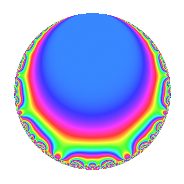# Properties

 Label 4020.2.q.gLevel 4020 Weight 2 Character orbit 4020.q Analytic conductor 32.100 Analytic rank 0 Dimension 2 CM No Inner twists 2

# Related objects

## Newspace parameters

 Level: $$N$$ = $$4020 = 2^{2} \cdot 3 \cdot 5 \cdot 67$$ Weight: $$k$$ = $$2$$ Character orbit: $$[\chi]$$ = 4020.q (of order $$3$$ and degree $$2$$)

## Newform invariants

 Self dual: No Analytic conductor: $$32.0998616126$$ Analytic rank: $$0$$ Dimension: $$2$$ Coefficient field: $$\Q(\sqrt{-3})$$ Coefficient ring: $$\Z[a_1, \ldots, a_{7}]$$ Coefficient ring index: $$1$$ Sato-Tate group: $\mathrm{SU}(2)[C_{3}]$

## $q$-expansion

Coefficients of the $$q$$-expansion are expressed in terms of a primitive root of unity $$\zeta_{6}$$. We also show the integral $$q$$-expansion of the trace form.

 $$f(q)$$ $$=$$ $$q$$ $$+ q^{3}$$ $$- q^{5}$$ $$-\zeta_{6} q^{7}$$ $$+ q^{9}$$ $$+O(q^{10})$$ $$q$$ $$+ q^{3}$$ $$- q^{5}$$ $$-\zeta_{6} q^{7}$$ $$+ q^{9}$$ $$+ 5 \zeta_{6} q^{11}$$ $$+ ( 1 - \zeta_{6} ) q^{13}$$ $$- q^{15}$$ $$+ ( 7 - 7 \zeta_{6} ) q^{17}$$ $$+ ( 5 - 5 \zeta_{6} ) q^{19}$$ $$-\zeta_{6} q^{21}$$ $$+ ( -9 + 9 \zeta_{6} ) q^{23}$$ $$+ q^{25}$$ $$+ q^{27}$$ $$+ 3 \zeta_{6} q^{29}$$ $$+ 7 \zeta_{6} q^{31}$$ $$+ 5 \zeta_{6} q^{33}$$ $$+ \zeta_{6} q^{35}$$ $$+ ( -7 + 7 \zeta_{6} ) q^{37}$$ $$+ ( 1 - \zeta_{6} ) q^{39}$$ $$+ 3 \zeta_{6} q^{41}$$ $$-8 q^{43}$$ $$- q^{45}$$ $$-7 \zeta_{6} q^{47}$$ $$+ ( 6 - 6 \zeta_{6} ) q^{49}$$ $$+ ( 7 - 7 \zeta_{6} ) q^{51}$$ $$+ 6 q^{53}$$ $$-5 \zeta_{6} q^{55}$$ $$+ ( 5 - 5 \zeta_{6} ) q^{57}$$ $$+ 4 q^{59}$$ $$+ ( 13 - 13 \zeta_{6} ) q^{61}$$ $$-\zeta_{6} q^{63}$$ $$+ ( -1 + \zeta_{6} ) q^{65}$$ $$+ ( 7 + 2 \zeta_{6} ) q^{67}$$ $$+ ( -9 + 9 \zeta_{6} ) q^{69}$$ $$-7 \zeta_{6} q^{71}$$ $$+ ( 5 - 5 \zeta_{6} ) q^{73}$$ $$+ q^{75}$$ $$+ ( 5 - 5 \zeta_{6} ) q^{77}$$ $$-13 \zeta_{6} q^{79}$$ $$+ q^{81}$$ $$+ ( -17 + 17 \zeta_{6} ) q^{83}$$ $$+ ( -7 + 7 \zeta_{6} ) q^{85}$$ $$+ 3 \zeta_{6} q^{87}$$ $$+ 18 q^{89}$$ $$- q^{91}$$ $$+ 7 \zeta_{6} q^{93}$$ $$+ ( -5 + 5 \zeta_{6} ) q^{95}$$ $$+ ( 1 - \zeta_{6} ) q^{97}$$ $$+ 5 \zeta_{6} q^{99}$$ $$+O(q^{100})$$ $$\operatorname{Tr}(f)(q)$$ $$=$$ $$2q$$ $$\mathstrut +\mathstrut 2q^{3}$$ $$\mathstrut -\mathstrut 2q^{5}$$ $$\mathstrut -\mathstrut q^{7}$$ $$\mathstrut +\mathstrut 2q^{9}$$ $$\mathstrut +\mathstrut O(q^{10})$$ $$2q$$ $$\mathstrut +\mathstrut 2q^{3}$$ $$\mathstrut -\mathstrut 2q^{5}$$ $$\mathstrut -\mathstrut q^{7}$$ $$\mathstrut +\mathstrut 2q^{9}$$ $$\mathstrut +\mathstrut 5q^{11}$$ $$\mathstrut +\mathstrut q^{13}$$ $$\mathstrut -\mathstrut 2q^{15}$$ $$\mathstrut +\mathstrut 7q^{17}$$ $$\mathstrut +\mathstrut 5q^{19}$$ $$\mathstrut -\mathstrut q^{21}$$ $$\mathstrut -\mathstrut 9q^{23}$$ $$\mathstrut +\mathstrut 2q^{25}$$ $$\mathstrut +\mathstrut 2q^{27}$$ $$\mathstrut +\mathstrut 3q^{29}$$ $$\mathstrut +\mathstrut 7q^{31}$$ $$\mathstrut +\mathstrut 5q^{33}$$ $$\mathstrut +\mathstrut q^{35}$$ $$\mathstrut -\mathstrut 7q^{37}$$ $$\mathstrut +\mathstrut q^{39}$$ $$\mathstrut +\mathstrut 3q^{41}$$ $$\mathstrut -\mathstrut 16q^{43}$$ $$\mathstrut -\mathstrut 2q^{45}$$ $$\mathstrut -\mathstrut 7q^{47}$$ $$\mathstrut +\mathstrut 6q^{49}$$ $$\mathstrut +\mathstrut 7q^{51}$$ $$\mathstrut +\mathstrut 12q^{53}$$ $$\mathstrut -\mathstrut 5q^{55}$$ $$\mathstrut +\mathstrut 5q^{57}$$ $$\mathstrut +\mathstrut 8q^{59}$$ $$\mathstrut +\mathstrut 13q^{61}$$ $$\mathstrut -\mathstrut q^{63}$$ $$\mathstrut -\mathstrut q^{65}$$ $$\mathstrut +\mathstrut 16q^{67}$$ $$\mathstrut -\mathstrut 9q^{69}$$ $$\mathstrut -\mathstrut 7q^{71}$$ $$\mathstrut +\mathstrut 5q^{73}$$ $$\mathstrut +\mathstrut 2q^{75}$$ $$\mathstrut +\mathstrut 5q^{77}$$ $$\mathstrut -\mathstrut 13q^{79}$$ $$\mathstrut +\mathstrut 2q^{81}$$ $$\mathstrut -\mathstrut 17q^{83}$$ $$\mathstrut -\mathstrut 7q^{85}$$ $$\mathstrut +\mathstrut 3q^{87}$$ $$\mathstrut +\mathstrut 36q^{89}$$ $$\mathstrut -\mathstrut 2q^{91}$$ $$\mathstrut +\mathstrut 7q^{93}$$ $$\mathstrut -\mathstrut 5q^{95}$$ $$\mathstrut +\mathstrut q^{97}$$ $$\mathstrut +\mathstrut 5q^{99}$$ $$\mathstrut +\mathstrut O(q^{100})$$

## Character Values

We give the values of $$\chi$$ on generators for $$\left(\mathbb{Z}/4020\mathbb{Z}\right)^\times$$.

 $$n$$ $$1141$$ $$2011$$ $$2681$$ $$3217$$ $$\chi(n)$$ $$-\zeta_{6}$$ $$1$$ $$1$$ $$1$$

## Embeddings

For each embedding $$\iota_m$$ of the coefficient field, the values $$\iota_m(a_n)$$ are shown below.

For more information on an embedded modular form you can click on its label.

Label $$\iota_m(\nu)$$ $$a_{2}$$ $$a_{3}$$ $$a_{4}$$ $$a_{5}$$ $$a_{6}$$ $$a_{7}$$ $$a_{8}$$ $$a_{9}$$ $$a_{10}$$
841.1
 0.5 − 0.866025i 0.5 + 0.866025i
0 1.00000 0 −1.00000 0 −0.500000 + 0.866025i 0 1.00000 0
3781.1 0 1.00000 0 −1.00000 0 −0.500000 0.866025i 0 1.00000 0
 $$n$$: e.g. 2-40 or 990-1000 Significant digits: Format: Complex embeddings Normalized embeddings Satake parameters Satake angles

## Inner twists

Char. orbit Parity Mult. Self Twist Proved
1.a Even 1 trivial yes
67.c Even 1 yes

## Hecke kernels

This newform can be constructed as the intersection of the kernels of the following linear operators acting on $$S_{2}^{\mathrm{new}}(4020, [\chi])$$:

 $$T_{7}^{2}$$ $$\mathstrut +\mathstrut T_{7}$$ $$\mathstrut +\mathstrut 1$$ $$T_{11}^{2}$$ $$\mathstrut -\mathstrut 5 T_{11}$$ $$\mathstrut +\mathstrut 25$$ $$T_{17}^{2}$$ $$\mathstrut -\mathstrut 7 T_{17}$$ $$\mathstrut +\mathstrut 49$$